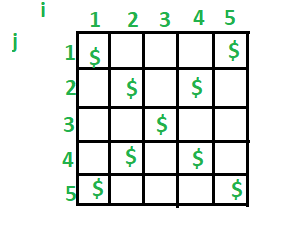# C++ Program to Print Cross or X Pattern

Given a number n, we need to print a X pattern of size n.

```Input : n = 3
Output :
\$ \$
\$
\$ \$

Input : n = 5
Output :
\$   \$
\$ \$
\$
\$ \$
\$   \$

Input : n = 4
Output :
\$  \$
\$\$
\$\$
\$  \$
```## Recommended: Please try your approach on {IDE} first, before moving on to the solution.

We need to print n rows and n columns. So we run two nested loops. The outer loop prints all rows one by one (runs for i = 1 to n). The inner loop (runs for j = 1 to n) runs all columns of current row. Now a row can contain spaces and ‘\$’. How do we decide where to put space and where ‘\$’.

For i = 1 : First and last column should contain ‘\$’
For i = 2 : Second and second last column should contain ‘\$’
In general, i-th and (n + 1 – i)-th columns should contain ‘\$’

 `// Program to make an X shape \$ pattern in c++ ` `#include ` `using` `namespace` `std; ` ` `  `int` `main() ` `{  ` `    ``// n denotes the number of lines in which  ` `    ``// we want to make X pattern ` `    ``int` `n;  ` `     `  `    ``// i denotes the number of rows ` `    ``cout << ``"Enter the value of n \n"``; ` `    ``cin >> n; ` ` `  `    ``// Print all rows one by one  ` `    ``for` `(``int` `i = 1; i <= n; i++) { ` ` `  `        ``// Print characters of current row ` `        ``for` `(``int` `j = 1; j <= n; j++)  ` `        ``{ ` `            ``// For i = 1, we print a '\$' only in  ` `            ``// first and last columns ` `            ``// For i = 2, we print a '\$' only in  ` `            ``// second and second last columns ` `            ``// In general, we print a '\$' only in  ` `            ``// i-th and n+1-i th columns ` ` `  `            ``if` `(j == i || j == (n + 1 - i)) ` `                ``cout << ``"\$"``;  ` `            ``else` `                ``cout << ``" "``; ` `        ``} ` `  `  `        ``// Print a newline before printing the ` `        ``// next row.  ` `        ``cout << endl; ` `    ``} ` `    ``return` `0; ` `} `My Personal Notes arrow_drop_upCheck out this Author's contributed articles.

If you like GeeksforGeeks and would like to contribute, you can also write an article using contribute.geeksforgeeks.org or mail your article to contribute@geeksforgeeks.org. See your article appearing on the GeeksforGeeks main page and help other Geeks.

Please Improve this article if you find anything incorrect by clicking on the "Improve Article" button below.